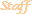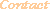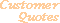Home Older Mathematica Items

Scientific Arts

# Mathematica Notebooks, Packages, and Other Examples

 LoanFunctions.m is a package containing functions that help in calculations of certain loan costs: for estimating home purchase costs and performing other loan calculations. This is a prototypical example of a self contained small application of the sort that Scientific Arts can easily deliver. It contains numerical and analytical functions, on-line documentation, floating palettes, and GUI calculators. Radar System Analysis Packages At Scientific Arts we have written a large collection of Mathematica functions that perform the calculations essential in the analysis of the performance of radar systems. A simple application of Mathematica string manipulation commands. ArrowExtended.m Package The ArrowExtended.m package extends some of the functionality of Mathematica's Graphics`Arrow` standard add-on package so that the HeadShape option can include Splines, Polygons with Splines, Plot, and ParametricPlot. In each case these may be multiplied by an arbitrary numerical scaling factor. Eye Diagram for Digital Communications In digital communications the "eye diagram" is used to visualize how the waveforms used to send multiple bits of data can potentially lead to errors in the interpretation of those bits. Certain pulse shapes are used specifically to alleviate this. One simple example of this is the "raised cosine" pulse shape. This is an example of an implementation of the eye diagram in Mathematica for the raised cosine pulse shape for Pulse Amplitude Modulation waveforms. Web Safe Colors Palettes Mathematica palettes and associated notebook for generating web safe colors. Stellar Structure and the Lane-Emden Function A discussion of the Lane-Emden function discussed in Chapter IV of "An Introduction to the Study of Stellar Structure" (Dover, New York, 1958) by S. Chandrasekhar. This function specifies the behavior of the equilibrium configuration of polytropic and isothermal gas spheres: stars. Maxwellian Probability Distribution Function The Maxwellian probability distribution function governs the distribution of molecular speeds in an ideal gas. Several methods for generating random numbers based on this distribution are described and coded. Example of Differential Equations for Chemical Kinetics Chemical reactions of a wide variety can be modeled with coupled (often nonlinear) differential equations. These describe the time evolution of the concentrations of the various chemical species: reactants, intermediaries, catalysts, and products. Such problems are quite simple to set up and solve with Mathematica. Single Electron Atom Wave Functions Computation (analytic and numerical) and visualization of single electron atom wave functions. Exploring a Data Set: Plotting with Error Bars Custom graphical visualization of a data set that describes the total interaction cross section for an elementary particle reaction. Mathematica in Quantum Field Theory Calculations: An Example A simple example of the use of Mathematica in the calculation of the one loop "fish" diagram in a quantum field theory. Building a Simple Ball and Stick Molecule Graphic from Graphics Primitives Often the visualization of data can be performed most conveniently by making use of Mathematica's graphics programming language directly. Here we do a rather simple exercise: produce a "ball and stick" graphic of a molecule. The molecule is specified in terms of the atoms that it is composed of, their coordinates in a Cartesian coordinate system, and the bonds between them. From Simple Tabular Formatting to Automatic Report Generation A simple example of the use of some Mathematica's tabular formatting and notebook manipulation commands to show how one can create functions that automatically generate reports. A Simple Analysis of Historical Sunspot Data Linear filtering in the time domain and low pass filtering in the frequency domain are used to smooth the time series that for the number of sunspots. This basic analysis gives along with a basic peak finding method determines the approximately 11 year sunspot cycle. Linear and Nonlinear Least Squares Fitting to Data An introductory exposition of the use of Mathematica's linear and nonlinear least squares fitting procedures to provide functional fits to data sets. Compilation and Packed Arrays An example of the use of Compile in a procedural program for the Mandelbrot set. Also included is a brief introduction to packed arrays. Arrow Axes A notebook showing the design of a function to add arrow heads to the axes of a graph.
 For further information on our services send email to info@scientificarts.com . Contents of this web site Copyright © 1999-2011, Scientific Arts, LLC.
 *Scientific Arts, LLC is not affiliated with Wolfram Research Inc. MATHEMATICA® a is a registered trademark of Wolfram Research, Inc.